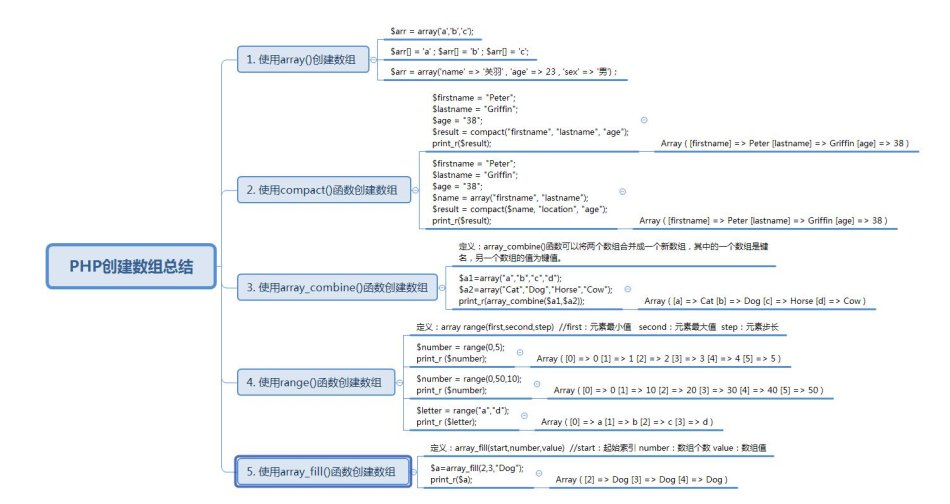• php创建数组的方法有：1、使用array()函数创建；2、使用compact()函数创建；3、使用array_combine()函数创建；4、使用range()函数创建；5、使用array_fill()函数创建。1、使用array()创建数组(推荐教程：mysql教程)...
php创建数组的方法有：1、使用array()函数创建；2、使用compact()函数创建；3、使用array_combine()函数创建；4、使用range()函数创建；5、使用array_fill()函数创建。1、使用array()创建数组(推荐教程：mysql教程)array()创建数组是我们在PHP开发过程中最常用到的一种方式，准确来说array()是一种结构而不是一个函数。示例1：$number = array(1,3,5,7,9);$color =array("red","blue","green");$student = array("name",17)示例2：$language = array(1=>"PHP",3=>"JAVA",4=>"C");$student = array("name"=>"张三","age"=>17)当然，数组里没有值也是允许的，即空数组：$result = array();2、使用compact()函数创建数组PHP中compact()函数可以将一个或多个变量转换为数组定义格式：array compact(var1,var2...)示例1：任何没有变量名与之对应的字符串都被略过。$firstname = "Peter";$lastname = "Griffin";$age = "38";$result = compact("firstname", "lastname", "age");print_r($result);输出结果：Array([firstname] => Peter[lastname] => Griffin[age] => 38)示例2：使用没有对应变量名的字符串，以及一个变量名数组$firstname = "Peter";$lastname = "Griffin";$age = "38";$name = array("firstname", "lastname");$result = compact($name, "location", "age");print_r($result);输出结果：Array([firstname] => Peter[lastname] => Griffin[age] => 38)3、使用array_combine()函数创建数组PHP中array_combine()函数可以将两个数组合并成一个新数组，其中的一个数组是键名，另一个数组的值为键值。定义格式：array array_combine(array1,array2)示例$a1=array("a","b","c","d");$a2=array("Cat","Dog","Horse","Cow");print_r(array_combine($a1,$a2));输出结果：Array ( [a] => Cat [b] => Dog [c] => Horse [d] => Cow )注意：使用array_combine()函数时，两个参数必须有相同数目的元素。4、使用range()函数创建数组定义格式：array range(first,second,step)first：元素最小值second：元素最大值step：元素步长下面是官方给的定义：该函数创建一个数组，包含从 first 到 second (包含 first 和 second)之间的整数或字符。如果 second 比 first 小，则返回反序的数组。理解起来比较吃力，我们直接看例子(本人就喜欢看有例子的教程)。示例1：$number = range(0,5);print_r ($number);输出结果：Array( => 0 => 1 => 2 => 3 => 4 => 5)示例2：$number = range(0,50,10);print_r ($number);输出结果：Array( => 0 => 10 => 20 => 30 => 40 => 50)示例3：$letter = range("a","d");print_r ($letter);输出结果：Array( => a => b => c => d)5、使用array_fill()函数创建数组array_fill()函数是用给定的值类填充数组定义格式：array_fill(start,number,value)start：起始索引number：数组个数value：数组值示例：$a=array_fill(2,3,"Dog");print_r($a);输出结果：Array (  => Dog  => Dog  => Dog )相关推荐：php培训
展开全文• php创建数组的方法有：1、使用array()函数创建；2、使用compact()函数创建；3、使用array_combine()函数创建；4、使用range()函数创建；5、使用array_fill()函数创建。1、使用array()创建数组(推荐教程：mysql教程)...
php创建数组的方法有：1、使用array()函数创建；2、使用compact()函数创建；3、使用array_combine()函数创建；4、使用range()函数创建；5、使用array_fill()函数创建。1、使用array()创建数组(推荐教程：mysql教程)array()创建数组是我们在PHP开发过程中最常用到的一种方式，准确来说array()是一种结构而不是一个函数。示例1：$number = array(1,3,5,7,9);$color =array("red","blue","green");$student = array("name",17)示例2：$language = array(1=>"PHP",3=>"JAVA",4=>"C");$student = array("name"=>"张三","age"=>17)当然，数组里没有值也是允许的，即空数组：$result = array();2、使用compact()函数创建数组PHP中compact()函数可以将一个或多个变量转换为数组定义格式：array compact(var1,var2...)示例1：任何没有变量名与之对应的字符串都被略过。$firstname = "Peter";$lastname = "Griffin";$age = "38";$result = compact("firstname", "lastname", "age");print_r($result);输出结果：Array([firstname] => Peter[lastname] => Griffin[age] => 38)示例2：使用没有对应变量名的字符串，以及一个变量名数组$firstname = "Peter";$lastname = "Griffin";$age = "38";$name = array("firstname", "lastname");$result = compact($name, "location", "age");print_r($result);输出结果：Array([firstname] => Peter[lastname] => Griffin[age] => 38)3、使用array_combine()函数创建数组PHP中array_combine()函数可以将两个数组合并成一个新数组，其中的一个数组是键名，另一个数组的值为键值。定义格式：array array_combine(array1,array2)示例$a1=array("a","b","c","d");$a2=array("Cat","Dog","Horse","Cow");print_r(array_combine($a1,$a2));输出结果：Array ( [a] => Cat [b] => Dog [c] => Horse [d] => Cow )注意：使用array_combine()函数时，两个参数必须有相同数目的元素。4、使用range()函数创建数组定义格式：array range(first,second,step)first：元素最小值second：元素最大值step：元素步长下面是官方给的定义：该函数创建一个数组，包含从 first 到 second (包含 first 和 second)之间的整数或字符。如果 second 比 first 小，则返回反序的数组。理解起来比较吃力，我们直接看例子(本人就喜欢看有例子的教程)。示例1：$number = range(0,5);print_r ($number);输出结果：Array( => 0 => 1 => 2 => 3 => 4 => 5)示例2：$number = range(0,50,10);print_r ($number);输出结果：Array( => 0 => 10 => 20 => 30 => 40 => 50)示例3：$letter = range("a","d");print_r ($letter);输出结果：Array( => a => b => c => d)5、使用array_fill()函数创建数组array_fill()函数是用给定的值类填充数组定义格式：array_fill(start,number,value)start：起始索引number：数组个数value：数组值示例：$a=array_fill(2,3,"Dog");print_r($a);输出结果：Array (  => Dog  => Dog  => Dog )相关推荐：php培训
展开全文• 在本文将数组的各种创建方式用PHP实例代码的方式分享给大家，感兴趣的朋友可以了解下看这篇文章之前相信大家都已经看过PHP中文手册关于数组这一节的讲解了，怎么样呢，看懂了多少？至少我第一次阅读文档时是一头雾水...
在本文将数组的各种创建方式用PHP实例代码的方式分享给大家，感兴趣的朋友可以了解下看这篇文章之前相信大家都已经看过PHP中文手册关于数组这一节的讲解了，怎么样呢，看懂了多少？至少我第一次阅读文档时是一头雾水，也许是因为在翻译的不够通俗易懂吧^_^！！这里UncleToo根据自己的经验，将数组的各种创建方式用PHP实例代码的方式分享给大家，希望对大家有些帮助(当然，PHP文档还是要多看的)1、使用array()创建数组array()创建数组是我们在PHP开发过程中最常用到的一种方式，准确来说array()是一种结构而不是一个函数。示例1：代码如下:<?php $number = array(1,3,5,7,9);$color =array("red","blue","green");$student = array("name",17)?>示例2：代码如下:<?php$language = array(1=>"PHP",3=>"JAVA",4=>"C");$student = array("name"=>"张三","age"=>17)?>当然，数组里没有值也是允许的，即空数组：代码如下:<?php$result = array();?>2、使用compact()函数创建数组PHP中compact()函数可以将一个或多个变量转换为数组定义格式：array compact(var1,var2...)示例1：任何没有变量名与之对应的字符串都被略过。代码如下:<?php $firstname = "Peter";$lastname = "Griffin";$age = "38";$result = compact("firstname", "lastname", "age");print_r($result);?>输出结果：代码如下:Array([firstname] => Peter[lastname] => Griffin[age] => 38)示例2：使用没有对应变量名的字符串，以及一个变量名数组代码如下:<?php$firstname = "Peter";$lastname = "Griffin";$age = "38";$name = array("firstname", "lastname");$result = compact($name, "location", "age");print_r($result);?>输出结果：代码如下:Array([firstname] => Peter[lastname] => Griffin[age] => 38)3、使用array_combine()函数创建数组PHP中array_combine()函数可以将两个数组合并成一个新数组，其中的一个数组是键名，另一个数组的值为键值。定义格式：array array_combine(array1,array2)示例代码如下:<?php $a1=array("a","b","c","d");$a2=array("Cat","Dog","Horse","Cow");print_r(array_combine($a1,$a2));?>输出结果：Array ( [a] => Cat [b] => Dog [c] => Horse [d] => Cow )注意：使用array_combine()函数时，两个参数必须有相同数目的元素。4、使用range()函数创建数组定义格式：array range(first,second,step)first：元素最小值second：元素最大值step：元素步长下面是官方给的定义：该函数创建一个数组，包含从 first 到 second (包含 first 和 second)之间的整数或字符。如果 second 比 first 小，则返回反序的数组。理解起来比较吃力，我们直接看例子(本人就喜欢看有例子的教程)。示例1：代码如下:$number = range(0,5);print_r ($number);?>输出结果：代码如下:Array( => 0 => 1 => 2 => 3 => 4 => 5)示例2：代码如下:<?php $number = range(0,50,10);print_r ($number);?>输出结果：代码如下:Array( => 0 => 10 => 20 => 30 => 40 => 50)示例3：代码如下:<?php $letter = range("a","d");print_r ($letter);?>输出结果：代码如下:Array( => a => b => c => d)5、使用array_fill()函数创建数组array_fill()函数是用给定的值类填充数组定义格式：array_fill(start,number,value)start：起始索引number：数组个数value：数组值示例：代码如下:<?php $a=array_fill(2,3,"Dog");print_r($a);?>输出结果：Array (  => Dog  => Dog  => Dog )
展开全文• 然而PHP创建数组的方法有哪些呢？在查阅资料并且网上收集整理总结了下：1. 使用array创建数组，是最常见和便捷的一个方式 :$arr = array('a','b','c');$arr[] = 'a' ; $arr[] = 'b' ;$arr[] = 'c...
在PHP以及其他程序语言程序设计中，经常会用到数组的创建，在前端开发中，js的数组创建可以由[]和new Array()直接创建和赋值。然而PHP创建数组的方法有哪些呢？在查阅资料并且网上收集整理总结了下：1. 使用array创建数组，是最常见和便捷的一个方式 :$arr = array('a','b','c');$arr[] = 'a' ; $arr[] = 'b' ;$arr[] = 'c';
$arr = array('name' => '关羽' , 'age' => 23 , 'sex' => '男')；2. 有时候需要创建一个长度为len的一个随机数组，所以就可以用range()： 定义：array range(first,second,step) //first：元素最小值 second：元素最大值 step：元素步长（要增加的量）$number = range(0,5);
print_r ($number); //打印：Array (  => 0  => 1  => 2  => 3  => 4  => 5 )$number = range(0,50,10);
print_r ($number); //打印：Array (  => 0  => 10  => 20  => 30  => 40  => 50 )$letter = range("a","d");
print_r ($letter); //打印：Array (  => a  => b  => c  => d ) 3.当然，在某些特定需求下，需要把某些变量存入数组，这些变量的变量名转换成键名，变量值转换成键值（前提：该变量存在）：$firstname = "Peter";
$lastname = "Griffin";$age = "38";
$result = compact("firstname", "lastname", "age"); print_r($result);

//打印： Array ( [firstname] => Peter [lastname] => Griffin [age] => 38 )

$firstname = "Peter";$lastname = "Griffin";
$age = "38";$name = array("firstname", "lastname");
$result = compact($name, "location", "age");
print_r($result); //打印：Array ( [firstname] => Peter [lastname] => Griffin [age] => 38 ) //由于$location变量不存在，所以存入不了数组4. 将两个数组合并为一个新的数组，第一个数组的值是新数组的键名，第二个数组里面的值是新数组的键值：定义：array_combine()函数可以将两个数组合并成一个新数组，其中的一个数组是键名，另一个数组的值为键值。 $a1=array("a","b","c","d");$a2=array("Cat","Dog","Horse","Cow");
print_r(array_combine($a1,$a2));

//打印：Array ( [a] => Cat [b] => Dog [c] => Horse [d] => Cow ) 5. 创建键值一样具有固定长度的数组：定义：array_fill(start,number,value)  //start：起始索引 number：值的个数 value：数组值$a=array_fill(2,3,"Dog"); print_r($a);

//打印：Array (  => Dog  => Dog  => Dog ) 
展开全文php array
• 在PHP程序设计中，经常会用到数组的创建，PHP创建数组的方法有哪些呢？在查阅相关资料并且网上收集整理总结如下使用array创建数组，是最常见和便捷的一个方式 :$arr = array('a','b','c');$arr[] = 'a' ; $arr[] = '... • 数组基本操作可以归纳为 增、删、改、查，需要留意的是哪些方法会对原数组产生影响...push()方法接收任意数量的参数，并将它们添加到数组末尾，返回数组的最新长度 letcolors=[];//创建一个数组 letcount=colors.... • 然而PHP创建数组的方法有哪些呢？在查阅资料并且网上收集整理总结了下： 1. 使用array创建数组，是最常见和便捷的一个方式 :$arr = array('a','b','c'); $arr[] = 'a' ;$arr[] = 'b...
• 数组基本操作可以归纳为 增、删、改、查，需要留意的是...push()方法接收任意数量的参数，并将它们添加到数组末尾，返回数组的最新长度 let colors = []; // 创建一个数组 let count = colors.push("red", "green"); /.js javascript
• 一、什么是数组？ 数组：数据的组合，每个数据都使用逗号分隔，数据可以是任何类型，数据顺序排列，可以重复。 二、数组的构成 1、索引（下标）：数组中的数据按照顺序...三、数组的创建方式 1、字面量创建 var arr...javascript
• 方法创建一个长度为 n 的数组对象，虽然长度 但仍无实际值 3. var 变量名 =[ 值1 , 值2 , 值3 , 值4 ,值5] 此方法创建一个数组对象 ，[ ] 内填写值 值与值之间逗号分隔 也可能只写一个[ ] var x = new ...javascript js
• Numpy库是现在机器学习，深度学习等人工智能和科学计算必不可少工具。其最核心数据结构就是Numpy数组...现在，我们就来看看有哪些方法可以快速创建你自己的数组。 将Python列表准换成Numpy数组 import Numpy ...Numpy
• 上一章咱们讲了数组的创建，接下来，咱们就看看数组应该怎么用，都有哪些方法，这些方法到底是做什么的,本文只介绍Array特有的方法，继承来自Object的方法不做赘述。contact顾名思义，这个方法是用来做合并的，但...javascript Array array
• 最可靠的两种 Array.isArray() (推荐) Array.isArray() 用于确定传递的值是否是一个 Array。如果对象是 Array，则为true;...问题：数组类型等内置类型，重写了toString方法，直接调用数组对象的方法，不在返回cla...js
• 本篇文章主要讲述ES5中的数组，包括数组两种创建方式，属性，以及9大类，总共23个操作方法...数组作为javascript中最常用的数据类型之一，掌握好数组的方法在日常的开发中是非常必要的，javascript中的数组相比其...
• 创建方式: 直接量 var a = [1, 2]构造函数: var b = new Array(1, 2) // 注意: 构造函数中只传一个参数会变成长度。数组APIvar push 添加元素：返回length，并把参数添加到数组中。pop 删除元素: 删除最后一位元素，...
• 数组的定义 数组是相同类型数据的有序集合 数组描述的是相同类型的若干个数据，按照一定的先后次序排列组合而成 其中，每一个数据称作一个数组元素...Java语言使用new操作符来创建数组，语法如下： dataType[] arrayjava jvm 算法 数据结构
• 数组去重包括es6的新方法我们调用一个数组的时候时候只做记录出现过哪些数，这个时候重复出现的就可以排除掉，例如通过摄像头拍摄的画面来记录多少人通过，重复拍到的人也只能算一个。这个时候就要排除掉重复...js es6 去重
• 前言: 在使用数组之前,我们想清楚一个问题:为什么要用数组？数组适用于哪些场合？ 想一下生活中的例子: 10 个...那什么简单的方法吗？答案是肯定的,让我们带着这些不解 let's go. 数组的创建: Java数组的创建java 数据结构 算法
• 面向对象概述面向对象(Object ...使用面向对象思维去开发程序，我们首先思考是这个系统中有哪些对象（事物），它们各自有什么属性（特征），又有什么方法（行为），这样一来，就可以把系统分解为一个一个...
• 1. 前言 数组真的是每天用了，但是有很多方法都是记不住，总是要...创建数组，怎么创建数组的 数组的构造方法Array有哪些方法？ES5 , ES6 ES6把类似数组对象转化成数组方法，ES5怎么把类似数组的对象转化成数组，原...
• ArrayList实现原理其实就是数组(动态数组)，ArrayList介绍及简单使用方法动态数组与一般数组有什么区别？与Java中的数组相比，ArrayList容量能动态地增长ArrayList效率怎么样？ArrayList不是线程安全，所以...
• --private Arrays() {}2_2、自己去创建工具类A、私有化构造方法B、写静态方法完成功能2_3、toString(数组)---返回一个String类型的数组内容2_3、sort(数组)--数组升序排序 3、基本数据类型包装类有哪些？...
• 有哪些方法？如何遍历？ 针对这些问题我在这里小结一下； 一、数组定义、特点、创建 1. 定义： 数组是一组数据集合，其表现形式就是内存中一段连续内存地址，数组名称其实就是连续内存地址首地址 2. 特点...javascript
• 第八章数组与字符串 微软IT学院郝全超 第八章...C#如何重新抛出异常举个简单的例子 如何自定义异常举例说明 完成课后题 内容回顾 数组的概念 声明和创建数组 通过索引来访问数组 复制数组 把数组作为方法参数 System
• 谈到检测JaveScript中数据类型，那么我们先了解一下JaveScript中数据有哪些类型 这里不考虑ES6新增第七种Symbol数据类型，它实例是唯一且不可改变类型值以及BigInt，他 是一种数字类型数据 目录JaveScript...javascript
• 这次给大家带来怎样用numpy找出数组里最大与最小值，用...可以使用一下方法：一、创建数组这样就可以获得一个array最大值和最小值了。并且可以利用np.where(np.max(a))来获得最大值，最小值行和列数。二、py...
• 这次给大家带来怎样用numpy找出数组里最大与最小值，用...可以使用一下方法：一、创建数组这样就可以获得一个array最大值和最小值了。并且可以利用np.where(np.max(a))来获得最大值，最小值行和列数。二、py...
• 之前的推文中，数模君跟大家介绍了数组的创建和运算，相信大家已经熟悉了数组的概念。那么在MATLAB中，可以对数组中的元素进行哪些操作呢？今天数模君就带大家了解一下数组操作的常用方法。其中，针对数组中元素的......